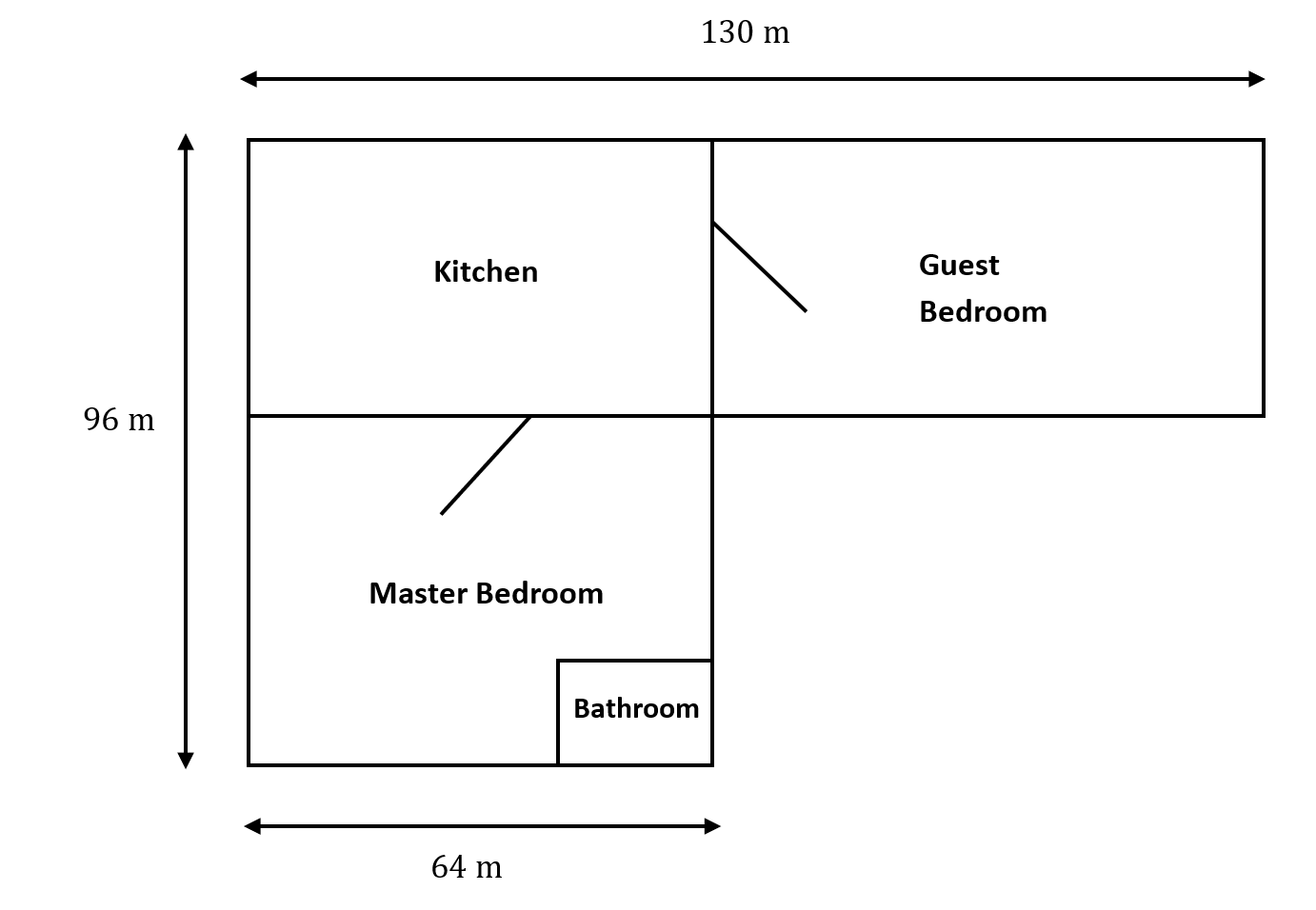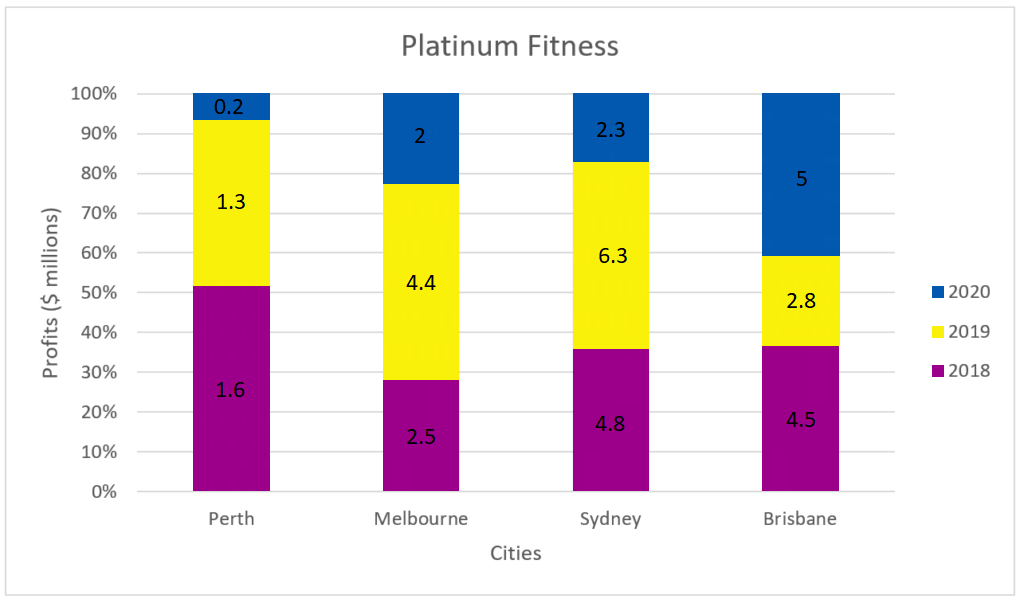# How to Ace UCAT Perimeter and Area or Chart and Graph Questions | Quantitative Reasoning Subtest

Need help acing UCAT? In this article, we'll show you how to answer Area and Perimeter & Chart and Graph questions for the Quantitative Reasoning subtest!

Do you get stressed out trying to solve perimeter and area questions for the quantitative reasoning subtest? Do you run out of time or get confused with chart and graph questions? Don’t worry, in this article, we going to show you how to ace these types of Quantitative Reasoning subtest questions.

## What common issues do UCAT students share in the Quantitative Reasoning subtest?

Students that sit the Quantitative subtest typically tend to struggle with the following:

• Time constraints. Students are unable to use the calculator at the UCAT examination centers effectively to maximize efficiency.
• Attention to detail. Students struggle with identifying key pieces of information and glazing over the irrelevant details to maximize efficiency.

Students may confuse formulas/steps when trying to solve the questions quickly. Training speed and accuracy together is only possible with practice under timed conditions!

Overall, the biggest struggle for students who sit this subtest is time management. Learning strategies and techniques which minimize time wastage and maximize efficiency will assist you in acing the exam!

## So how do I fix this?

One strategy to improve your time management is to systematically break down complex questions into basic steps. This makes your thinking clearer and workflow faster. By going through some difficult sample questions, this article will demonstrate by example exactly how you can do this yourself with fully worked solutions.

## Want to see where you’re at with the UCAT?## What problem types will I have in the QR subtest?

The following problem types are explored in the Quantitative subtest:

• Chart / Graph reading
• Percentages
• Averages: means, modes and medians
• Ratios and proportionality
• Rates and Converting rates
• Perimeters, areas and volumes

## Perimeters, areas and volumes

The diagram (not to scale) below outlines the floor plan for a collection of new apartments:• The bathroom makes up $25\%$ of the combined area of both the kitchen and the guest bedroom.
• The cost of covering the floors with carpeting is priced at $\6$ per square metre, or $\9.50$ per square metre for tiling.

### Question 1:

If the area of the kitchen is $390 \text{ m}^2$ smaller than the area of the master bedroom, what is the area of the kitchen, in square metres?

1. $2877 \text{ m}^2$
2. $3267 \text{ m}^2$
3. $5754 \text{ m}^2$
4. $6144 \text{ m}^2$

### Step 1:

The tricky part of this question is that finding the width of the kitchen and master bedroom is not given so it’s important to find the total area of both the kitchen and master bedroom first:

$96×64=6144 \text{ m}^2$
$∴K+M=6144$

### Step 2:

The next part of this question is using the information provided in the question, which is that the kitchen is $390 \text{ m}^2$ smaller than the master bedroom.

$∴M=K+390$

### Step 3:

The last part is to solve the two equations generated from the question simultaneously:

$K+M=6144$
$M=K+390$
$∴K+K+390=6144$
$2K=5754$
$K=2877 \text{ m}^2$

### Question 2:

If the perimeter of the master bedroom (including the bathroom) is $390\text{ m}$, what is the total area of the bathroom and guest bedroom?

1. $845 \text{ m}^2$
2. $963 \text{ m}^2$
3. $1274 \text{ m}^2$
4. $2561 \text{ m}^2$
5. $6895 \text{ m}^2$

### Step 1:

Use the information about the perimeter to determine the dimensions of the master bedroom. Let $x$ be the length of the left side of the master bedroom.

Then, we have

$2x+2(64)=268$
$2x=140$
$x=70$

### Step 2:

Find the area of the bathroom first, which would require the combined area of the kitchen and the guest bedroom.

Since the left side length of the master bedroom and the left side length of the kitchen sum to $96 \text{ m}$, we know the left side length of the kitchen is $96-70=26 \text{ m}$.

Hence, the combined area of the kitchen and the guest bedroom is $26×130=3380 \text{ m}^2$ .

The area of the bathroom is thus $25\%×3380=845\text{ m}^2$.

### Step 3:

Find the area of the guest bedroom. From Step 2 the left side length of the guest bedroom is the same as the left side length of the kitchen, which is $26$.

The top side of the guest bedroom has length $130-64=66 \text{ m}$ and so the area of the guest bedroom is $66×26=1716 \text{ m}^2$.

### Step 4:

Find the total area.

The combined area of the bathroom and the guest bedroom is $845+1716=2561 \text{ m}^2$.

### Question 3:

If the length of the left side of the kitchen is $50\text{ m}$, what is the length of the left side of the bathroom?

1. $5 \text{ m}$
2. $5 \text{ m}$
3. $6 \text{ m}$
4. $0 \text{ m}$
5. Can’t Tell

### Solution:

There is actually not enough information to determine the answer to this. It’s not stated that all the rectangles are in perfect similarity with each other nor are any one of the dimensions of the bathroom provided. Hence, the solution is Can’t tell.

A common mistake would be to do $50×25\%$, but this is not necessarily true. Just because the area of the kitchen is $25\%$ of the combined area of the kitchen and guest bedroom, does not mean the side length of the bathroom is $25\%$ of the side length of the kitchen/guest bedroom.

### Question 4:

The owners of the apartment decide to place carpeting across the entire apartment except for the bathroom and decide to place tiling across the bathroom. What is the total cost to do this if the area of the kitchen was $3264 \text{ m}^2$?

1. $\7335.00$
2. $\54195.5$
3. $\62861.25$
4. $\72806.25$
5. Can’t Tell

### Step 1:

Use the area of the kitchen to find the length of the left side of the kitchen.

Since left side length $×64=3264 \text{ m}^2$, the left side length must be $51\text{ m}$.

### Step 2:

Find the area of the kitchen and the guest bedroom. Since the side length is $51$, the area must be $51×130=6630 \text{ m}^2$.

### Step 3:

Use the combined area of the kitchen and the guest bedroom to find the area of the bathroom.

The area of the bathroom is $25 \%×6630=1657.5$.

### Step 4:

Using the side length of the kitchen, find the side length of the master bedroom and find its area (less the area of the bathroom).

The side length of the master bedroom is $96-51=45 \text{ m}$ .

The combined area of the master bedroom and the bathroom is $45×64=2880 \text{ m}^2$

Hence the area of the master bedroom alone is $2880-1657.5=1222.5 \text{ m}^2$.

### Step 5:

Use the areas found in Step 1 to 4 to find the total cost.

The carpeting for the kitchen and the guest bedroom will cost $6630 \text{ m}^2×\6 / \text{ m}^2=\39780$

The carpeting for the master bedroom will cost $1222.5 \text{ m}^2 ×\6/ \text{ m}^2=\7335$

The tiling for the bathroom will cost $1657.5 \text{ m}^2×\9.50/ \text{ m}^2 =\15746.25$

Hence, the total cost is $39780+7335+15746.25=\62861.25$

## Chart / Graph reading

The chart below provides information on a large gym organization and the profits it made from different cities in Australia.Profit figures are in millions of dollars. For example, $2.3$ would be referring to $2.3$ million dollars.

### Question 1:

Which city made the highest total profit for all three years?

1. Perth
2. Melbourne
3. Sydney
4. Brisbane
5. Can’t Tell

### Solution: C

To save time for this question, use the eyeball method or quick basic arithmetic to determine that Sydney is the city which made the most profit.

### Question 2:

How much profit did Platinum Fitness make in Australia in 2020?

1. $9.5$ million
2. $12.6$ million
3. $14.88$ million
4. $13.4$ million
5. $37.7$ million

### Solution: A

This would be the sum of the figures in grey (which represent the profit made in 2020 in each city): $0.2+2+2.3+5=9.5$ million.

### Question 3:

What is the percentage change in profit from 2018 to 2019 in Sydney?

1. $-63.49%$
2. $-31.25%$
3. $31\%$
4. $25\%$
5. $49\%$

### Solution: D

$\text{Percentage Change}=\frac{\text{New profit}-\text{Old Profit}} {\text{Old profit}} ×100$
$=(6.3-4.8)/4.8×100$
$=31.25\%$

### Question 4:

Express the profit made by Melbourne in 2018 as a percentage compared to the total profit made by all cities in 2018.

1. $63\%$
2. $66\%$
3. $05\%$
4. $73\%$
5. $09\%$

### Step 1:

Find the total profit.

The profit made by Melbourne in 2018 is: $2.5$ million .

The total profit made by all cities in 2018 is: $1.6+2.5+4.8+4.5=13.4$ million.

### Step 2:

Calculate Melbourne’s profit as a percentage of the total.

The percentage is $\frac{2.5}{13.4}×100=18.66\%$

## Need help with acing your UCAT?